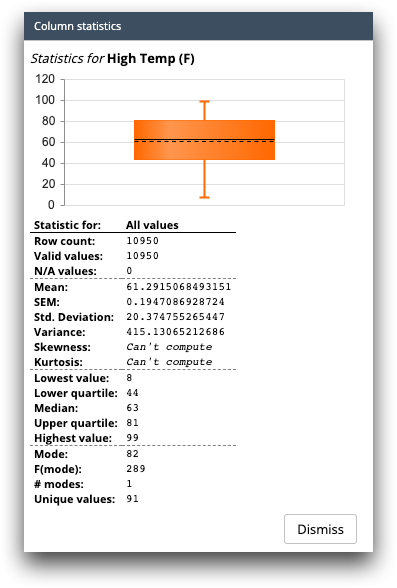# Basic statistics

The Basic statistics view provides summary statistics about the data in a selected column.

The Basic statistics view in the results pane is accessed by right-clicking a cell in the grid and then selecting Quick analysis of [COLUMN_LABEL] > Basic statistics from the menu.

If a find expression is in effect in the grid, then two columns are shown: one giving the statistics for the entire table, and one giving the statistics for only the highlighted subset of the table. If you change the find expression in the grid, the second column will update automatically.

Note: In this topic, [COLUMN_LABEL] represents the label of the column in which the selected cell is located.Figure: Basic statistics view in the results pane of the TRS and the MLW

The following information is available in the Basic statistics view:

Row count
The total number of values (both N/A and non-N/A) in the selected column.
Valid values
The number of non-N/A values located in the selected column.
N/A values
The number of N/A values located in the selected column.
Mean
The mean of the values in the selected column. (numeric columns only)
• If the column contains N/A values, those rows are ignored (i.e., the result is the average of the non-N/A values).
• If the column contains only N/A values, the result is N/A.
Std. Deviation
The standard deviation of the values in the selected column. (numeric columns only)
• If the column contains N/A values, those rows are ignored (i.e., the result is the standard deviation of the non-N/A values).
• If the column contains only N/A values, the result is N/A.
Lowest value
The minimum value located in the selected column.
Median
The median value of the selected column.
Highest value
The maximum value located in the selected column.
Mode
The mode of the values in the selected column.
# modes
The number of times the mode value appears in the selected column.
Unique values
The number of unique values in the selected column.
• If the column contains N/A values, those rows are ignored (i.e., the result is the number of unique non-N/A values).
• If the column contains only N/A values, the result is 0.